﻿ PipeLay > Software Components & Operation > Defining a Line > Line Subcomponents > Cable > Cable Data Inputs

# Cable Data Inputs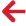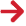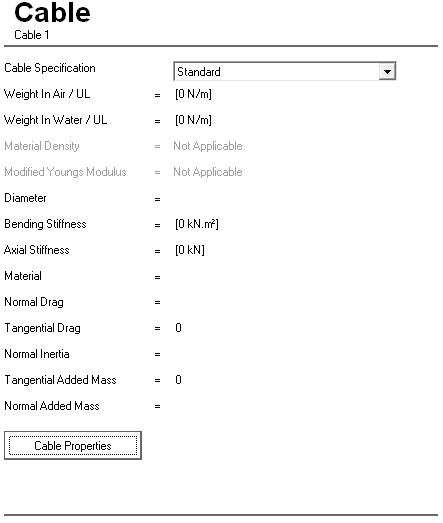Input Description Cable Specification: This drop-down list is used to select the format in which the cable properties are specified. The options are Standard (the default) and Direct. The Standard format references a Material component and is based on a solid cross section, whereas the Direct format requires you to specify properties directly and assumes a stranded section. Cable Properties Click on this button to display the Cable Properties dialog. The layout of this dialog is dependent on the selected Cable Specification. See the proceeding tables for a detailed description of this dialog.

Cable - Standard Specification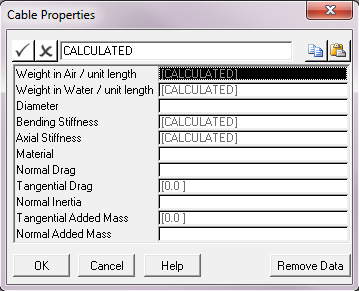Input Description Weight in Air / Unit Length: The dry weight of the cable. This may be either a number or the word “CALCULATED”. The default is “CALCULATED” which means that the value of this input is computed internally using the values you specify for cable Diameter, material Mass Density and Acceleration due to Gravity. However, you can override this computation, and input a value directly in this text box. Units: [N/m] or [lb/ft] Weight in Water / Unit Length: The submerged weight of the cable. This may be either a number or the word “CALCULATED”. The default is “CALCULATED” which means that the value of this input is computed internally using the values you specify for cable Diameter, material Mass Density, Acceleration due to Gravity  and Water Density. However, you can override this computation, and input a value directly in this text box. Units: [N/m] or [lb/ft] Diameter: The diameter of the cable. Units: [mm] or [in] Bending Stiffness: The bending stiffness of the cable. This may be either a number or the word “CALCULATED”. The default is “CALCULATED” which means that the value of this input is computed internally using the values you specify for cable diameter and material Young’s Modulus. However, you can override this computation, and input a value directly for this text box. Units: [kNm2] or [kips.ft2] Axial Stiffness: The axial stiffness of the cable. This may be either a number or the word “CALCULATED”. The default is “CALCULATED” which means that the value of this input is computed internally using the values you specify for cable diameter and material Young’s Modulus. However, you can override this computation, and input a value directly for this text box. Units: [kN] or [kips] Material: A drop-down list that allows the selection of a Material component from all those already defined in the project. Normal Drag: The Morison’s Eq. drag coefficient for the direction normal to the cable, denoted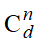. Tangential Drag: The Morison’s Eq. drag coefficient for the direction tangential to the section, denoted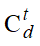. This defaults to zero. Normal Inertia: The Morison’s Eq. inertia coefficient for the direction normal to the cable, denoted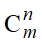. Tangential Added Mass: The Morison’s Eq. added mass coefficient in the direction tangential to the section, denoted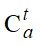. This input is optional and defaults to zero if omitted. Normal Added Mass: The Morison’s Eq. added mass coefficient in the direction normal to the section, denoted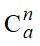. This input is optional and defaults to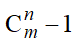if omitted.

Cable - Direct Specification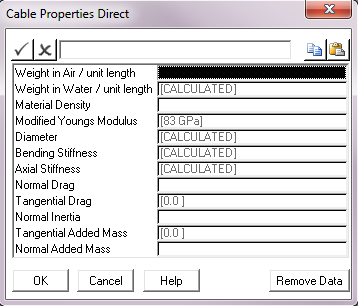Input Description Weight in Air / Unit Length: The dry weight of the cable. This is a mandatory input as it is used to determine other characteristics of the cable. Units: [N/m] or [lb/ft] Weight in Water / Unit Length: The submerged weight of the cable. This may be either a number or the word “CALCULATED”. The default is “CALCULATED” which means that the value of this input is computed internally using the values you specify for cable Weight in Air per Unit Length, Acceleration due to Gravity, Material Density and Water Density. However, you can override this computation, and input a value directly in this text box. Units: [N/m] or [lb/ft] Material Density: The mass density (ρ) of the cable material. This is a mandatory input as it is used to determine other characteristics of the cable. Units: [kg/m3] or [slugs/ft3] Modified Young’s Modulus: The modified Young’s Modulus (E) of the cable material. This is mandatory input and it defaults to 83 GPa metric or 12,000 ksi imperial. Units: [GPa] or [ksi] Diameter: The diameter of the cable. This may be either a number or the word “CALCULATED”. The default is “CALCULATED” which means that the value of this input is computed internally using the values you specify for cable Weight in Air per Unit Length, Acceleration due to Gravity and Material Density. However, you can override this computation, and input a value directly in this text box. Note that if you do specify an override value then it will only affect the contact diameter for interaction with supports and the drag diameter for hydrodynamic loadings. The effective diameter for stress calculations will always be based on the internal computation. Units: [mm] or [in] Bending Stiffness: The bending stiffness of the cable. This may be either a number or the word “CALCULATED”. The default is “CALCULATED” which means that the value of this input is computed internally using the values you specify for the cable Weight in Air per Unit Length, Acceleration due to Gravity, Material Density and Modified Young’s Modulus. However, you can override this computation, and input a value directly for this text box. Units: [kNm2] or [kips.ft2] Axial Stiffness: The axial stiffness of the cable. This may be either a number or the word “CALCULATED”. The default is “CALCULATED” which means that the value of this input is computed internally using the values you specify for the cable Weight in Air per Unit Length, Acceleration due to Gravity, Material Density and Modified Young’s Modulus. However, you can override this computation, and input a value directly for this text box. Units: [kN] or [kips] Normal Drag: The Morison’s Eq. drag coefficient for the direction normal to the cable, denoted. Tangential Drag: The Morison’s Eq. drag coefficient for the direction tangential to the section, denoted. This defaults to zero. Normal Inertia: The Morison’s Eq. inertia coefficient for the direction normal to the cable, denoted. Tangential Added Mass: The Morison’s Eq. added mass coefficient in the direction tangential to the section, denoted. This input is optional and defaults to zero if omitted. Normal Added Mass: The Morison’s Eq. added mass coefficient in the direction normal to the section, denoted. This input is optional and defaults toif omitted.